### 4.2.2.1 NEWTON Algorithm for Optimization

The NEWTON iteration scheme offers a simple but very powerful root-search algorithm with a quadratic convergence property, if the initial guess is located within a confidence interval (domain) near the root. Hence the NEWTON iteration scheme provides a local root search for a function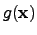and a local optimization strategy forif this function represents a spatial derivative of an objective function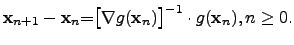(4.17)

The NEWTON iteration method can be applied for the optimization problem (4.1), where the minimum/maximum search of the score functionis equivalent to the root search of the spatial derivative of the score function if the function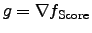substituted to (4.17). Hence, the optimization as a root search reads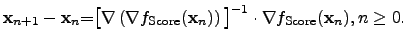(4.18)

In (4.17) and (4.18)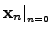represents the used-defined initial value,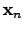is the current value of the NEWTON iteration, and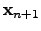is the next value which is improved by the local derivative for the objective function. An additional disadvantage of this method is the requirement of the second derivative of the objective function. If higher dimensional parameter spaces have to be considered, the computational effort can be enormous compared to the nominal number of parameter evaluations. To improve the convergence property the NEWTON optimization algorithm line searches are used. If the curvature of the function is positive, the optimum found is a local minimum, and otherwise a maximum.

Stefan Holzer 2007-11-19